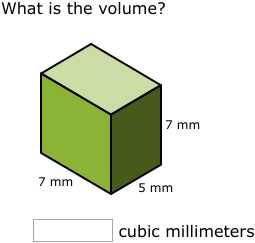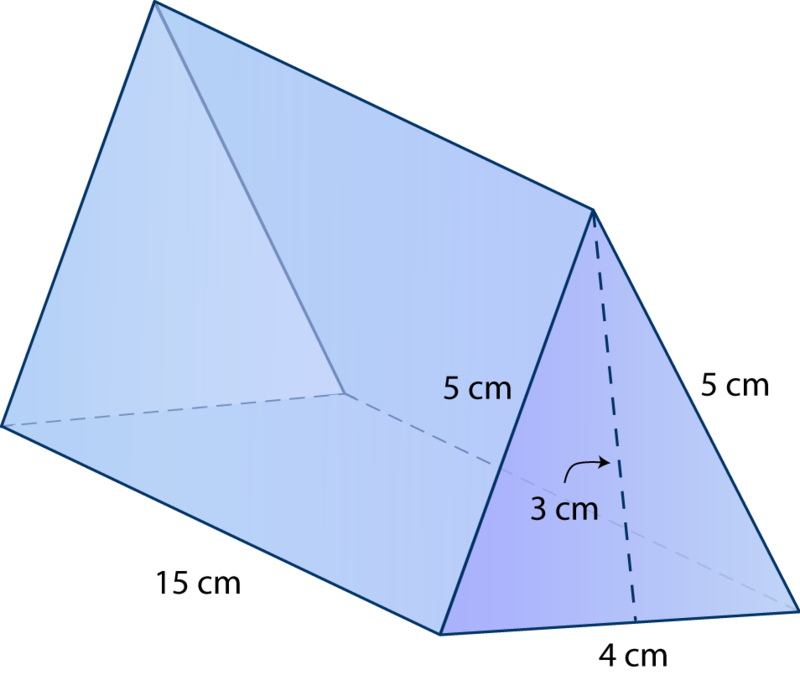Fractions
Algebra
Probability
Percentages
SA and V

Simplify

100/155

20/31

### 100

y x 5= 35

What is the value of y

y=7

### 100

Mike has 20 marbles.

3 green, 6 red, 4 blue, 2 purple, and the rest are pink.

How many are pink?

5 are pink

### 100

Mike has 20 marbles.

3 green, 6 red, 4 blue, 1 purple,and 6 pink.

What is the percent of red?

30%

### 100

What is the formula of calculating the SA of a triangle.

b x h ÷ 2

5×2/7

1 and 3/7

### 200

y×4=36

What is the value of y

y=9

### 200

Jeff has 120 coloured counters in a bag, there are red, blue, green and pink counters. 15 are red, 20 are blue, 35 are green, 30 are pink and 20 are purple.

What are the chances of him pulling out a pink and blue in fraction simplified

The chance of Jeff pulling out a pink and blue is 5/12.

8% of 25

2

### 200

How do you calculate the volume of a cube?

SxSxS

2/15 + 3/17=

79/255

x+13=18

y×15=75

y÷x=w

w=15

### 300

Steve has 200 cubes in a bag. In the bag he has 100 red cubes, 22 yellow cubes, 42 pink cubes and the rest are orange. How many orange cubes does Steve have? Put it in a fraction.

6% of 85

5.1

### 3007 x 5 = 35

35 x 7 = 245 mm3

### 400

Change the mix number into an improper fraction then find the missing number.

4 and 2/5 = ___ = 242/____

4 and 2/5 = 22/5= 242/55

### 400

y x 32= 384

What is the value of y

12

### 400

Mr. and Mrs. Stones just got 4 new dogs. They bought them 620 toys for all the dogs. 200 toys were balls, 374 were bones and the rest were animal stuffies. How many animal stuffies did the stones buy for their dogs. Show in fraction and percent.

5% of 3.14159

0.1570795

### 400222cm2

### 500

1/3 + 5/6 + 1/2 + 10/11 -5/3 =

60/66 = 10/11

### 500

y x 70=2240

What is the value of y?

y=32

### 500

There were 20 sharks at a beach. There were 250 kids all together and when the sharks attacked 130 kids were OK and the rest were injured. How many kids were injured show in fraction,decimal,and percent